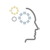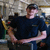## Puzzle – Find the number?Tags:
COBOL
Microsoft Excel
Programming Languages
VB
This is not a question & I am not sure if this is a proper forum. So I will let the site moderator decide. I recently came across a nice quiz which, I think, supports/encourages developing programming skills. So ... here is the puzzle. Find the lowest number with the following characteristics: When divided by 10 the remainder is 9 When divided by 9 the remainder is 8 When divided by 8 the remainder is 7 When divided by 7 the remainder is 6 When divided by 6 the remainder is 5 When divided by 5 the remainder is 4 When divided by 4 the remainder is 3 When divided by 3 the remainder is 2 When divided by 2 the remainder is 1 I used Excel with VB code to get the answer. It will be interesting to know how others solve it by using different programming languages. Good luck.

Thanks. We'll let you know when a new response is added.

Good old fashion paper and pencil (actually a pen ) got the answer of <b>2519</b>.
The simplest way is to find the LCM (Least Common Multiple) of the numbers 2, 3, 4, 5, 6, 7, 8 and 9, then subtract 1 from it. The LCM of 2, 3, 4, 5, 6, 7, 8 and 9 is 2520, Therefore, the answer is 2519.
Good Luck!
-Flame

*********************

public class FindTheNumber {

public static void main(String[] args) {
new FindTheNumber().findNumber();
}

void findNumber() {
int number = 10;
int div;
boolean foundTheNumber = false;
while (true) {
for (div = 2; div < 10; div++) {
if (rightNumber(number, div)) {
foundTheNumber = true;
for (int d = div; d >= 2; d–) {
if (rightNumber(number, d)) {
} else {
foundTheNumber = false;
break;
}
}
} else {
foundTheNumber = false;
break;
}
}
if (foundTheNumber && div == 10) {
System.out.println(“The Number is : ” + number );
System.exit(0);
} else {
number++;
}
}
}

boolean rightNumber(int number, int divider) {
if (number % divider == divider – 1) {
return true;
} else {
return false;
}
}
}

if you want to change it into LCM, just change “divider – 1” in rightNumber() method with “0”.
anyone have a better logic?
-Hendi

## Discuss This Question: 8 Replies

Thanks. We'll let you know when a new response is added.
•Hi SbElectric, Thanks for sharing this; I don't think there's anything wrong with the community helping to hone each other's skills. I'll have to try this later in Excel with VB as you mentioned. I'd love to see how other members figure this one out. Feel free to post your methods here!Community Moderator
report
•Flame, Going old school with the pencil and paper. Nice Sean
report
•Amazing, Flame. I used a brute force algorithm, and got the same answer.
report
•I used the MOD function with a For statement in VBA to find the same answer. Dustin
report
• Well ... it is nice to know that so many people got the number by different methods. That was my thought of seeing how others solve it. Initially, I also used brute force method (like Carlosdl) & then developed the simple Excel/VB program. Flame reminded the LCM process - which I totally forgot since the high school days! I was not aware that LCM function is available in Excel. I now know and used it --- so simper to use. I noticed Hendi used Java & RoadDust used MOD function. Is it possible to share the code - this will keep us informed of various techniques. I am copying my Excel/VB code for others to critiques. I set up Excel/VB to populate the number and the corresponding remainder values (from Row 10 and onwards). Then I compared the remainder pattern with “987654321” to check if the number is found. I do realize this is not the most elegant programming code! Sub DisplayNumber() ' Ctrl + Shft+ N is the shortcut to Run this macro ' Initial analysis reveals that the number must end with 9 ' Also start with 89 & then increment by 90 so that remainder ' by dividing with 9 will be 8. This is just to save excessive display ' You can start with 9 & then increment by 10 X = 89 For I = 10 To 100 Cells(I, 1) = X Y = 10 For J = 2 To 10 C = Int(X / Y) R = X - (C * Y) Cells(I, J) = R Y = Y - 1 Next J ' Form the pattern & compare with 9876... Cells(I, 11) = Cells(I, 2) & Cells(I, 3) & Cells(I, 4) & Cells(I, 5) & Cells(I, 6) & Cells(I, 7) & Cells(I, 8) & Cells(I, 9) & Cells(I, 10) If (Cells(I, 11) = "987654321") Then Cells(I, 12) = "Result" X = X + 90 Next I End Sub
report
•Nice, This was my first algorith (Oracle PL/SQL), just to check Flame's answer ( :-) ):
```create or replace PROCEDURE test IS
found boolean := false;
theNumber number := 9;
BEGIN
if mod(theNumber,10) = 9 then
if mod(theNumber,9) = 8 then
if mod(theNumber,8) = 7 then
if mod(theNumber,7) = 6 then
if mod(theNumber,6) = 5 then
if mod(theNumber,5) = 4 then
if mod(theNumber,4) = 3 then
if mod(theNumber,3) = 2 then
if mod(theNumber,2) = 1 then
dbms_output.put_line('The number is: '||theNumber);
found := true;
end if;
end if;
end if;
end if;
end if;
end if;
end if;
end if;
end if;
theNumber := theNumber + 10;
exit when theNumber > 100000;		-- Just in case
end loop;
END;```
Then I changed it to a shorter one:
```	while not found loop
for i in 2 .. 9 loop
if mod(theNumber,i) != i-1 then
exit;
elsif i = 9 then
dbms_output.put_line('The number is: '||theNumber);
found := true;
end if;
end loop;
theNumber := theNumber + 10;
exit when theNumber > 100000;		-- Just in case
end loop;```
report
•Sorry it took so long to respond, here is the code that I used to figure the same number. The MOD function returns just the remainder. For i = 1 To 10000 If i Mod 10 = 9 And i Mod 9 = 8 And i Mod 8 = 7 And i Mod 7 = 6 And i Mod 6 = 5 And i Mod 5 = 4 And i Mod 4 = 3 And i Mod 3 = 2 And i Mod 2 = 1 Then MsgBox i End If i = i + 1 Next Dustin
report
•A somewhat obvious speed enhancement to any of the brute force algorithms is that the first condition ("divided by 10 the remainder is 9") means that the last digit must be a "9". Ergo, any loop can be of the form: for i = 9 to 9999 step 10 -- use your favorite language syntax here And then, of course, you don't need to execute the "X mod 10 = 9" test. Further, since 9 is odd, you can skip the "X mod 2 = 1" test as well. Last bit of tuning is to recognize that the modulo function is more likely to succeed for false answers for small modulus values than for large modulus values, thus the optimum series is "for modulus = 8 downto 3". Resulting in (pseudo code):
```integer i
integer m
boolean bfound
for i = 9 to 9999 step 10
bfound = true
for m = 8 downto 3 step -1
if (i mod m) != (m-1) then
bfound = false
exit for    ' exit inner loop on first modulus test failure
end if
next ' m
if bfound then
msgbox i
exit for
end if
next ' i```
report